# Multilingual Wiki DocumentationYou are currently using syntax.In an attempt to improve PlantUML documentation...
Please do not use this website for your own diagrams.
You can click here and use the online server here for your own documentation.## Mathématiques

Vous pouvez utiliser les notations AsciiMath ou JLaTeXMath dans PlantUML:

@startuml :$int_0^1f(x)dx$; :$x^2+y_1+z_12^34$; note right Essayer aussi: $d/dxf(x)=lim_(h->0)(f(x+h)-f(x))/h$ <latex>P(y|\mathbf{x}) \mbox{ ou } f(\mathbf{x})+\epsilon</latex> end note @enduml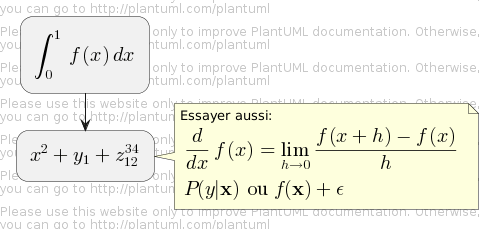ou encore:

@startuml Bob -> Alice : Peux-tu résoudre: $ax^2+bx+c=0$ Alice --> Bob: $x = (-b+-sqrt(b^2-4ac))/(2a)$ @endumlWARNING
This translation need to be updated.
WARNING

## Maths

Within PlantUML, you can use AsciiMath notation:

@startuml :$int_0^1f(x)dx$; :$x^2+y_1+z_12^34$; note right Try also $d/dxf(x)=lim_(h->0)(f(x+h)-f(x))/h$ $P(y|bb"x") or f(bb"x")+epsilon$ end note @enduml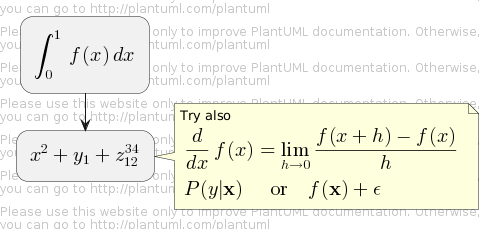or JLaTeXMath notation:

@startuml :<latex>\int_0^1f(x)dx</latex>; :<latex>x^2+y_1+z_{12}^{34}</latex>; note right Try also <latex>\dfrac{d}{dx}f(x)=\lim\limits_{h \to 0}\dfrac{f(x+h)-f(x)}{h}</latex> <latex>P(y|\mathbf{x}) \mbox{ or } f(\mathbf{x})+\epsilon</latex> end note @enduml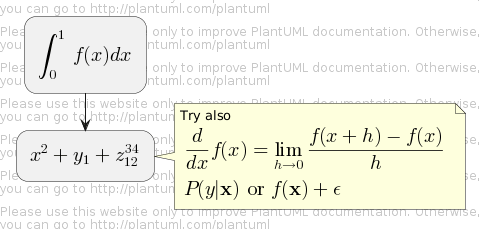Here is another example:

@startuml Bob -> Alice : Can you solve: $ax^2+bx+c=0$ Alice --> Bob: $x = (-b+-sqrt(b^2-4ac))/(2a)$ @enduml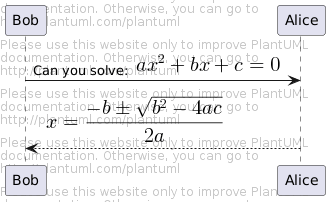## Diagramme indépendant

Il est possible d'utiliser @startmath/@endmath pour créer des formules AsciiMath.

@startmath f(t)=(a_0)/2 + sum_(n=1)^ooa_ncos((npit)/L)+sum_(n=1)^oo b_n\ sin((npit)/L) @endmath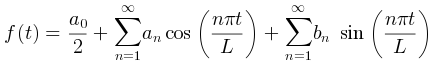Ou bien utiliser @startlatex/@endlatex pour créer des formules JLaTeXMath.

@startlatex \sum_{i=0}^{n-1} (a_i + b_i^2) @endlatex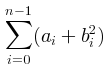## Standalone diagram

You can also use @startmath/@endmath to create standalone AsciiMath formula.

@startmath f(t)=(a_0)/2 + sum_(n=1)^ooa_ncos((npit)/L)+sum_(n=1)^oo b_n\ sin((npit)/L) @endmathOr use @startlatex/@endlatex to create standalone JLaTeXMath formula.

@startlatex \sum_{i=0}^{n-1} (a_i + b_i^2) @endlatex## Comment cela fonctionne ?

Pour dessiner ces formules, PlantUML utilise deux projets OpenSource:

• AsciiMath qui convertit la notation AsciiMath vers une expression LaTeX.
• JLatexMath qui dessine une formule mathématique écrite en LaTeX. JLaTeXMath est le meilleur projet Java pour dessiner du code LaTeX.

ASCIIMathTeXImg.js est suffisamment petit pour être intégré dans la distribution standard de PlantUML.

PlantUML relies on the Java Scripting API (specifically: new ScriptEngineManager().getEngineByName("JavaScript");) to load a JavaScript engine and execute JavaScript code. Java 8 includes a JavaScript engine called Nashorn but it was deprecated in Java 11.

If you are using AsciiMath in Java 11 you see the following warnings:

Warning: Nashorn engine is planned to be removed from a future JDK release

Nashorn was removed in Java 15. Fortunately, you can use the GraalVM JavaScript Engine instead by adding the following dependencies:

<dependency>
<groupId>org.graalvm.js</groupId>
<artifactId>js</artifactId>
<version>20.2.0</version>
</dependency>
<dependency>
<groupId>org.graalvm.js</groupId>
<artifactId>js-scriptengine</artifactId>
<version>20.2.0</version>
</dependency>


You can even use the GraalVM JavaScript Engine in Java 11 to get rid of the warning messages.

Comme JLatexMath est plus gros, vous devez le télécharger séparément, puis extraire les 4 fichiers (batik-all-1.7.jar, jlatexmath-minimal-1.0.3.jar, jlm_cyrillic.jar et jlm_greek.jar) dans le même répertoire que PlantUML.jar.

## How is this working?

To draw those formulas, PlantUML uses two open source projects:

• AsciiMath that converts AsciiMath notation to LaTeX expression;
• JLatexMath that displays mathematical formulas written in LaTeX. JLaTeXMath is the best Java library to display LaTeX code.

ASCIIMathTeXImg.js is small enough to be integrated into PlantUML standard distribution.

PlantUML relies on the Java Scripting API (specifically: new ScriptEngineManager().getEngineByName("JavaScript");) to load a JavaScript engine and execute JavaScript code. Java 8 includes a JavaScript engine called Nashorn but it was deprecated in Java 11.

If you are using AsciiMath in Java 11 you see the following warnings:

Warning: Nashorn engine is planned to be removed from a future JDK release

Nashorn was removed in Java 15. Fortunately, you can use the GraalVM JavaScript Engine instead by adding the following dependencies:

<dependency>
<groupId>org.graalvm.js</groupId>
<artifactId>js</artifactId>
<version>20.2.0</version>
</dependency>
<dependency>
<groupId>org.graalvm.js</groupId>
<artifactId>js-scriptengine</artifactId>
<version>20.2.0</version>
</dependency>


You can even use the GraalVM JavaScript Engine in Java 11 to get rid of the warning messages.

Since JLatexMath is bigger, you have to download it separately, then unzip the 4 jar files (batik-all-1.7.jar, jlatexmath-minimal-1.0.3.jar, jlm_cyrillic.jar and jlm_greek.jar) in the same folder as PlantUML.jar.

Please report any bugs to plantuml@gmail.com or here.
This website is still in beta testing.